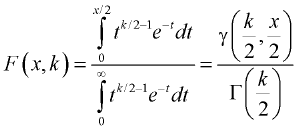Contents - Index

CHI_SQUARE

CHI_SQUARE(x,k) returns the value of the cumulative distribution function F(x,k) for the argument value x and k degrees of freedom.  The CHI_SQUARE cumulative distribution function is formally defined aswhere g is the lower incomplete Gamma function and G is the Gamma function.  The  CHI_SQUARE function is further defined in Abramowitz and Stegun, "Handbook of Mathematical Functions", Dover Publications, 10th printing, 1972.